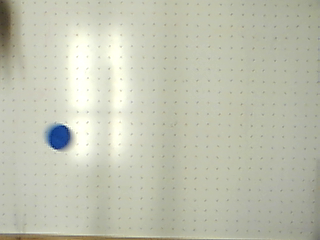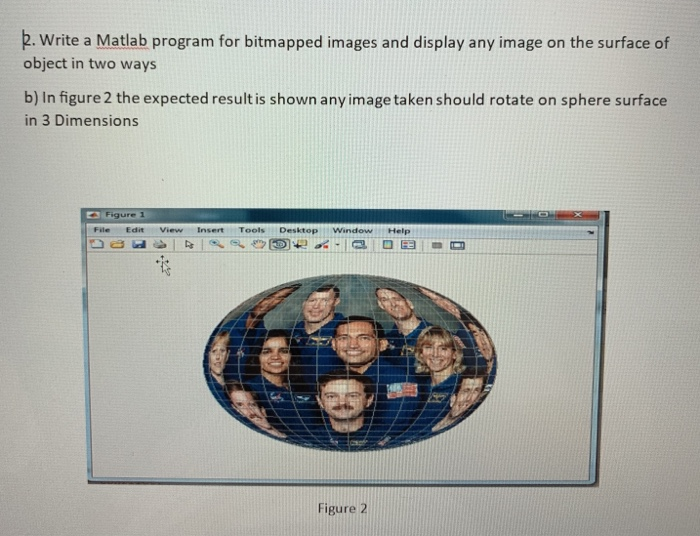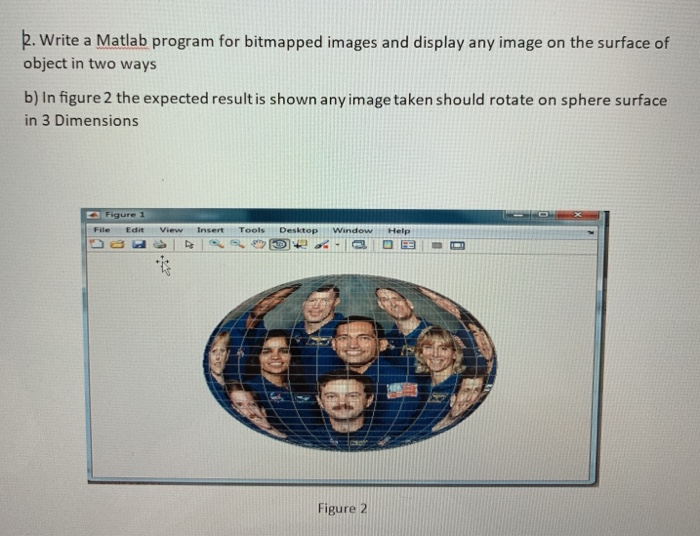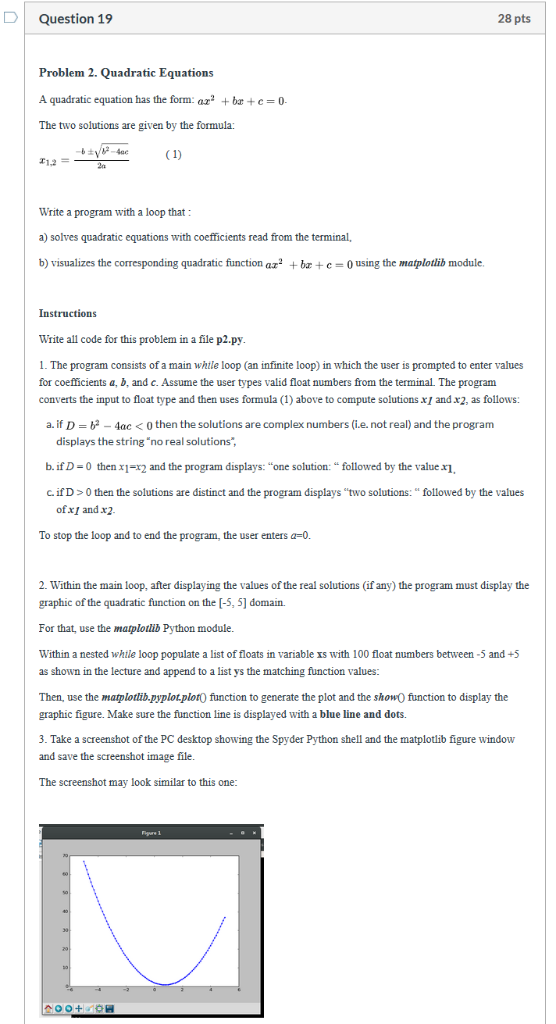# Given an image of a puck, write a program to get a white outline around the blue object. Save and...

Given an image of a puck, write a program to get a white outline around the blue object.
Save and display the image with the outline. The outline should be approximately similar
to the test case outputs shown below. Hint: Use binary image.

LANGUAGE : MATLAB ~~~~~ MATLAB ~~~~~~~~~MATLABimshow(I)

//convert to binary image

BW=im2bw(I);

imshow(BW)

//determine row and column

dim=size(BW)

col=round(dim(2)/2)-90;

row=min(find(BW(:,col)))

imshow(I)

hold on;

plot(boundary(:,2),boundary(:,1),'w','Linewidth',3);

##### Add Answer of: Given an image of a puck, write a program to get a white outline around the blue object. Save and...
Similar Homework Help Questions
• ### 2. Write a Matlab program for bitmapped images and display any image on the surface of object in ...2. Write a Matlab program for bitmapped images and display any image on the surface of object in two ways b) In figure 2 the expected result is shown any image taken should rotate on sphere surface in 3 Dimensions File Edit View InsertTools Desktop Window Help Figure 2 2. Write a Matlab program for bitmapped images and display any image on the surface of object in two ways b) In figure 2 the expected result is shown any image...

• ### P, write a Matlab program for bitmapped images and display any image on the surface of object in ...p, write a Matlab program for bitmapped images and display any image on the surface of object in two ways b) In figure 2 the expected result is shown any image taken should rotate on sphere surface in 3 Dimensions File Edit View Insert Tools Desktop Window Help Figure 2 p, write a Matlab program for bitmapped images and display any image on the surface of object in two ways b) In figure 2 the expected result is shown any...

• ### Write and test a MIPS assembly language program .... ?? Please I need it today

Write and test a MIPS assembly language program to read a text file containing only decimalintegers and sort them in descending order. The program should do the following:¦ Open a text file and read its content into an array of characters. The array should be limited to1000 characters. MARS provides the system calls for opening and reading from a text file.¦ Traverse the array character by character. Convert each decimal string into binary. A decimalstring consists of one or multiple...

• ### Using basic c++ write 2 separate codes for this assignment. Program #1 Write a program that...

Using basic c++ write 2 separate codes for this assignment. Program #1 Write a program that calculates the average of a group of test scores, where the lowest score in the group is dropped. It should use the following functions. • void getScore() should ask the user for a test score, store it in the reference parameter variable, and validate it. This function should be called by the main once for each of the five scores to be entered. •...

• ### Trees Traversals Please write a Java program to traverse a binary tree in in-order and pre-order, and to plot a binar...

Trees Traversals Please write a Java program to traverse a binary tree in in-order and pre-order, and to plot a binary tree into a 2-dimensional array. You may write many recursive methods for this project. You are not allowed to use any existing Java classes such as ArrayList or Vector or Tree. Please stop your program if the user enters 0 as the tree selection. Your program must run the following Test Case 1 plus two more test cases to...

• ### Write this program in C : ( NOT C++ )

Caesar Cipher ProjectOne of the oldest known encryption techniques is the Caesar cipher, attributed to Julius Caesar. It involves replacing each letter in a message with another letterthat is a fixed number of positions later in the alphabet. This number of positions is called the key of the cipher. If the replacement would go past the letter Z (orz for lowercase letters), the cipher “wraps around” to the beginning of the alphabet. For example, if the key is 2, then...

• ### In c++ The iron-puzzle.ppm image is a puzzle; it contains an image of something famous, howeve...

In c++ The iron-puzzle.ppm image is a puzzle; it contains an image of something famous, however the image has been distorted. The famous object is in the red values, however the red values have all been divided by 10, so they are too small by a factor of 10. The blue and green values are all just meaningless random values ("noise") added to obscure the real image. If you were to create a grayscale image out of just the red...

• ### DQuestion 19 28 pts Problem 2. Quadratic Equations A quadratic equation has the form: a bc0 The two solutions are given by the formula: 2a Write a program with a loop that a) solves quadratic equatio...DQuestion 19 28 pts Problem 2. Quadratic Equations A quadratic equation has the form: a bc0 The two solutions are given by the formula: 2a Write a program with a loop that a) solves quadratic equations with coefficients read from the terminal, b) visualizes the corresponding quadratic function az2 brc0using the matplotlib module. Instructions Write all code for this problem in a file p2.py 1. The program consists of a main whtle loop (an infinite loop) in which the user...

• ### writing a C++ program

Objectives• Apply structured and modular design principles to write programs that meet written specifications and requirements.• Develop a pseudo-code design using appropriate program structure (sequence, selection, repetition and nesting) to solve a given programming problem.• Use appropriate selection and repetition statements to implement the design.• Create user-defined functions to implement a modular design.• Use appropriate parameter passing mechanisms for passing data into and getting data back from functions.• Use ostream and iomanip formatting manipulators to display tabulated data.• Design and...

• ### Help me with this java program

Use JCreator to write and test a Java program that meets the requirements below.In this lab, you'll write a program to work with loops and arrays.Write code to display a dialog box with text "Part One" and an OK button.Create an array of doubles called grades; set the array size to 4.Prompt the user to enter four quiz grades, which are to be stored in the array. Use the Scanner class to read the user's response. More specifically, ask the...

Need Online Homework Help?